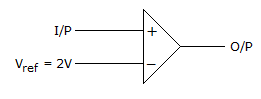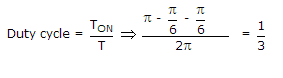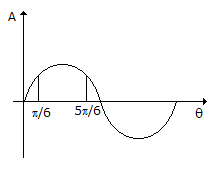# Electronics and Communication Engineering - Analog Electronics - Discussion

4.

If the input to the ideal comparator shown in the figure is a sinusoidal signal of 8 V (peak to peak) without any DC component, then the output of the comparator has a duty cycle of[A]. 1/2 [B]. 1/3 [C]. 1/6 [D]. 1/12

Explanation:Hima said: (Jul 19, 2014) How that pi/6 will come in the formula?

 Gaya3 said: (Aug 8, 2014) What is the impact of Vref in the prob?

 Geeta said: (Sep 10, 2014) How that pi-pi/6-pi/6 will come in the problem?

 Prajakta said: (Sep 17, 2014) How that pi/6 will come in formula?

 Bhanu said: (Oct 11, 2014) Same doubt. Why should we take pi/6?

 Sourabh Nath said: (Nov 14, 2014) Since Vref=2V, so the comparator can be assumed to set it as a threshold voltage. Now let us the compare the phase with the amplitude. When voltage exceeds 2V, phase is just pi/6. Again after reaching the peak value it returns to pi/6 at 5*pi/6 step. So the time t which it exceeds the threshold can be considered the ON time and the rest as OFF. Thus duty cycle = (pi-pi/6-pi/6) /pi which gives 1/3. Since frequency is constant. So phase is linearly proportional to time. i.e duty cycle = Ton/Toff satisfies the above expression.

 Vaibhav said: (Jan 14, 2015) Please explain with more details.

 Haritha Vangala said: (Jan 22, 2015) In this the total time period is 2pi, so (Ton+Toff) = 2pi. We know that duty cycle = Ton/(Ton+Toff). Now we should find Ton period i.e., here it is given that p-p voltage is 8v. So for +ve half cycle the amplitude is (0 to 4) we and phase is (0 to pi) right! It is given that ref voltage is 2v. So when ever the voltage crosses ref voltage comparator comes to ON state. At 2v the phase is pi/6 (mark 2 volts in the above wave plot and draw a horizontal line towards wave. That point shows the phase on theta axis). So phase is pi/6. Therefore the Ton is (pi- (pi/6+pi/6) ) which gives us the duty cycle as 1/3.

 Unknown said: (Feb 4, 2015) How can you tell that the total time period is 2*pi ? In the question it is not mentioned.

 Vaibhav said: (Feb 19, 2015) I still don't get how pi/6 comes, as it says 8v p-p so for +ve half cycle peak voltage is 4v so phase is pi/2, and for ref volt 2v the phase should be pi/4 not pi/6. And for -ve half cycle, does the comparator remain off? Why?

 Sagar said: (Mar 16, 2015) How at 2v phase is pi/6?

 Mani Kumar said: (Mar 31, 2015) In general the form of sinusoidal signal is vmsin(x), where vm is peak voltage. Given p-p is 8V, so vm=4v; so signal is 4sin(x) the period of sin wave is 2pi. equate 4sin(x) with 2 we get x=pi/6, 5pi/6; i.e sin(pi-pi/6) is also sin(pi/6), so on time is (pi-(2pi/6)). Duty cycle = (4pi/6)/2pi = 1/3.

 Umair said: (Apr 1, 2015) Great explanation, thanks to all for the elaboration.

 Darshith S P said: (Jul 21, 2015) As per me at 4V(+ve peak) the value of X-axis is PI/2, then at 2V the value of X-axis will become PI/4 & 3PI/4. If so then the duty cycle will become 1/4. i.e. 25% duty cycle. Please anyone confirm with this doubt.

 Chenelyn Cedron said: (Aug 1, 2016) First, Duty Cycle is the ratio of the amount of time the device is on/conducting to the total period of the device. The comparator will only be on if the input reaches 2 volts and higher. But as the input voltage goes down again beyond 2 volts, the comparator is off. This whole cycle takes 2π units so the period is 2π. To get the total time the comparator is on, use this formula: integral_from{π/6} to {5π/6} 1dx. Divide that to the period 2π. That's how you will get (1/3).

 Faisal Saleem said: (Oct 11, 2016) For those who wants to know about Pi/6. Vm = 4V as Vpp = 8V. Vref = V = 2V. As V = Vm Sin(X) So, 2 = 4Sin(X). Sin(X) = 0.5. X = Sin Inverse (0.5). X = 30 or Pi/6.

 Sirelaw said: (Oct 11, 2016) I didn't know about this topic before referring here but with the elaborate explanations, I understand it better now. Thank yo all.

 Lokesh said: (Nov 14, 2016) Is anyone tells the output of comparator irrespective of the duty cycle? I mean general comparator output.

 Dee said: (Mar 4, 2017) Thanks @Haritha and @Mani Kumar.

 Sangi said: (May 6, 2017) It's very helpful, thanks @Mani Kumar.

 Kanna said: (Aug 4, 2017) Please tell me how we got 5π/6?

 Lakshmishree said: (Mar 19, 2018) Great explanations, thank you all.

 Mona said: (Dec 4, 2018) Thank you all for explaining this.

 Mona said: (Dec 5, 2018) Why 5pi/6=? Explanation is; The total half cycle is of π. 2v is a reference so as per waveform projection will be at equal distance from 0 and 180° now first projection is at π/6 and total is π So,second projection =π-π/6 = 6π-π/6 = 5 π/6.

 Kalyan said: (Jan 23, 2019) Thanks all for explaining it.

 Darshan said: (Jul 21, 2019) Thanks all for the explanation.